JEE  >  Test: Straight Lines- 2

# Test: Straight Lines- 2

Test Description

## 25 Questions MCQ Test Mathematics (Maths) Class 11 | Test: Straight Lines- 2

Test: Straight Lines- 2 for JEE 2023 is part of Mathematics (Maths) Class 11 preparation. The Test: Straight Lines- 2 questions and answers have been prepared according to the JEE exam syllabus.The Test: Straight Lines- 2 MCQs are made for JEE 2023 Exam. Find important definitions, questions, notes, meanings, examples, exercises, MCQs and online tests for Test: Straight Lines- 2 below.
Solutions of Test: Straight Lines- 2 questions in English are available as part of our Mathematics (Maths) Class 11 for JEE & Test: Straight Lines- 2 solutions in Hindi for Mathematics (Maths) Class 11 course. Download more important topics, notes, lectures and mock test series for JEE Exam by signing up for free. Attempt Test: Straight Lines- 2 | 25 questions in 25 minutes | Mock test for JEE preparation | Free important questions MCQ to study Mathematics (Maths) Class 11 for JEE Exam | Download free PDF with solutions
 1 Crore+ students have signed up on EduRev. Have you?
Test: Straight Lines- 2 - Question 1

### The lines x + (k – 1) y + 1 = 0 and 2x+k2y−1 = 0 are at right angles if

Test: Straight Lines- 2 - Question 2

### A line is equally inclined to the axis and the length of perpendicular from the origin upon the line is √2. A possible equation of the line is

Detailed Solution for Test: Straight Lines- 2 - Question 2

Since the line is equally inclined the slope of the line should be -1, because it makes 135o in the positive direction of the X axis
This implies the equation of the line is y= -x +c
i.e; x+y - c =0
distance of the line from the origin is given as √2
Therefore √2 = |c|/(√12+12)
This implies c = 2
Hence the equation of the line is x+y=2

Test: Straight Lines- 2 - Question 3

### The lines y = mx , y + 2x = 0 , y = 2x + λ and y = - mx + λ form a rhombus if m =

Detailed Solution for Test: Straight Lines- 2 - Question 3

Given Lines:-
L1 : y=mx
L2: y+2x=0
L3 : y=2x+k
L4 : y+mx=k
To form a rhombus, opposite sides must be parallel to each other.
∵ Slopes of parallel lines are always equal
L1∥L3
L2 ∥ L4

Therefore,m = 2

Test: Straight Lines- 2 - Question 4

Two points (a , 0) and (0 , b) are joined by a straight line. Another point on this line is

Detailed Solution for Test: Straight Lines- 2 - Question 4

Equation of line x/a + y/b = 1
Given that (a,0) and (0,b) lie on a straight line.
We know that a straight line is represented by y = mx + c
Substituting co-ordinates in equation, we get
(1) 0 = am+c
(2) b=a(0)+c = c
⇒ m = −b/a, c = b
∴ Equation of line is ay=ab−bx
Substituting options we see that (3a,−2b) lies on this line.

Test: Straight Lines- 2 - Question 5

The coordinates of the foot of perpendicular from (0 , 0) upon the line x + y = 2 are

Detailed Solution for Test: Straight Lines- 2 - Question 5

Let the perpendicular line of x+y=2 is y−x=λ
It passes through (0,0), then λ=0
∴y−x=0
The point of intersection of y−x=0 and x+y=2 is (1,1), which is the required coordinates.

Test: Straight Lines- 2 - Question 6

The area of the triangle whose sides are along the lines x = 0 , y = 0 and 4x + 5y = 20 is

Test: Straight Lines- 2 - Question 7

A line is drawn through the points (3 , 4) and (5 , 6) . If the is extended to a point whose ordinate is – 1, then the abscissa of that point is

Detailed Solution for Test: Straight Lines- 2 - Question 7

Equation of line is (y-4)/(x-3) = (6-4)/(5-3)
y - 4 = x - 3
=> x - y + 1 = 0
Let abscissa of the point is a
:. (a, -1) should satisfy the equation
:. a- (-1) + 1 = 0
a = - 2

Test: Straight Lines- 2 - Question 8

The line x + y – 6 = 0 is the right bisector of the segment [PQ]. If P is the point (4, 3) , then the point Q is

Detailed Solution for Test: Straight Lines- 2 - Question 8

Given, the line equation : x+y−6=0 .....(i)
Co-ordinates of P are (4,3)
Let the co-ordinates of Q be (x,y)
Now, the slope of the given line is
y = 6-x
slope m = -1
So, the slope of PQ will be −1/m
​[As the product of slopes of perpendicular lines is −1]
Slope of PQ = -1/(-1) = 1
R lies on x + y - 6 = 0
(a+4)/2 + (b+3)/2 - 6 = 0
=> a + 4 + b + 3 - 12 = 0
=> a + b - 5 = 0.......(1)
Slope of LM = slope of PQ = -1
= -(1) * (b-3)/(a-4) = -1
= (b-3)/(a-4) = 1
= b-3 = a-4
=> a-b-1 = 0...............(2)
Solving (1) and (2), we get
a = 3, b = 2

Test: Straight Lines- 2 - Question 9

The acute angle between the lines ax + by + c = 0 and (a + b)x = (a – b)y, a ≠ b, is

Detailed Solution for Test: Straight Lines- 2 - Question 9

ax + by + c = 0 and (a + b)x = (a – b)y
m1 = -a/b,    m2 = (a+b)/(a-b)
tanx = [(m1-m2)/(1+m1×m2)]
=> {(-a/b)- (a+b)/(a-b)}/{1+(-a/b)[(a+b)/(a-b)]}
=> {-a2+ab-ab-b2}/{b(a-b)} * {ba-b2-a2-ab}/{b(a-b)}
=> (-a2-b2)/{1/(-a2-b2)
tanx = 1
x = tan-1(1)
Angle = 45o

Test: Straight Lines- 2 - Question 10

The lines x + 2y – 3 = 0, 2x + y – 3 = 0 and the line l are concurrent. If the line I passes through the origin, then its equation is

Detailed Solution for Test: Straight Lines- 2 - Question 10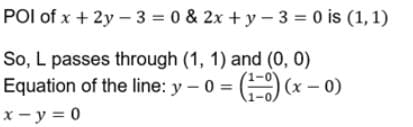Test: Straight Lines- 2 - Question 11

The triangle formed by the lines x + y = 1, 2x + 3y – 6 = 0 and 4x – y + 4 = 0 lies in

Detailed Solution for Test: Straight Lines- 2 - Question 11

We have,

x+y=1......(1)

2x+3y=6......(2)

4x−y=−4......(3)

From equation (1) and (2) to and we get,

(x+y=1)×2

2x+3y=6

2x+2y=2

2x+3y=6

On subtracting and we get,

y=4 put in (1) and we get,

2x+2y=2

2x+2(4)=2

2x+8=2

2x=−6

x=−3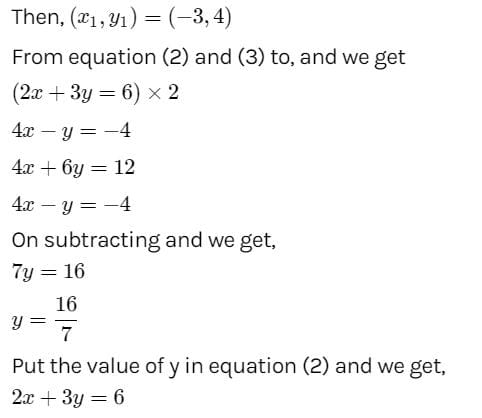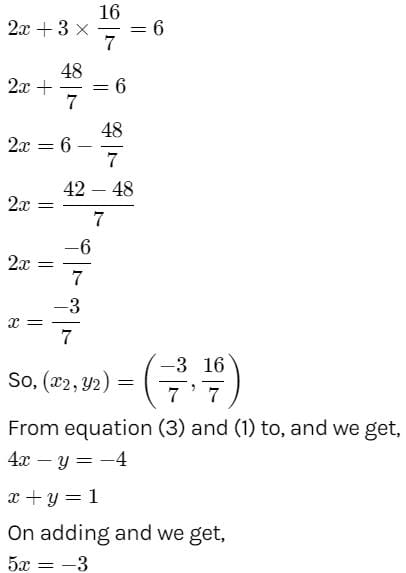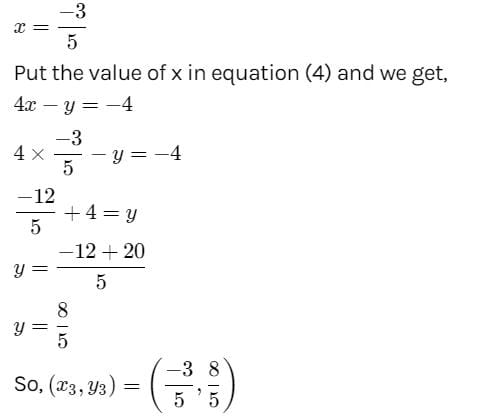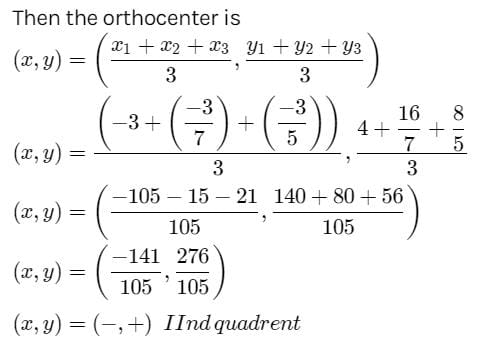Test: Straight Lines- 2 - Question 12

The area of triangle formed by the lines y = x, y = 2x and y = 3x + 4 is

Detailed Solution for Test: Straight Lines- 2 - Question 12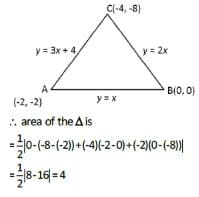Test: Straight Lines- 2 - Question 13

If the points representing the complex numbers - 4 +3i , 2 – 3i and 0 + π are collinear , then the value of p is

Detailed Solution for Test: Straight Lines- 2 - Question 13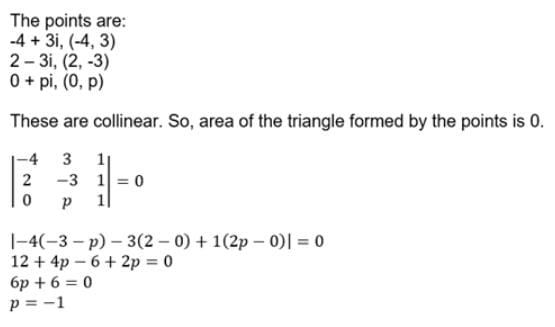Test: Straight Lines- 2 - Question 14

The lines ix + my + n = 0 , mx + ny + l = 0 and nx + ly +m = 0 are concurrent if

Detailed Solution for Test: Straight Lines- 2 - Question 14

Suppose we have three straight lines whose equations are:
a₁x + b₁y + c₁ = 0,
a₂x + b₂y + c₂ = 0
a₃x + b₃y + c₃ = 0.
These lines are said to be concurrent if the following condition holds:
Determinant of
a₁     b₁     c₁
a₂     b₂     c₂   =  0
a₃     b₃     c₃
Now
l       m     n
m     n      l     =   0
n      l      m
l(nm - l²) - m(m² - nl) + n(ml - n²) = 0
lmn - l³ - m³ + lmn + lmn - n³ = 0
l³ + m³ + n³ = 3lmn
this condition true if an only if
l + m + n = 0

Test: Straight Lines- 2 - Question 15

The equations of the lines through (- 1 , - 1) and making angles of 450 with the line x + y = 0 are

Test: Straight Lines- 2 - Question 16

Given the 4 lines with equations x + 2y – 3 = 0, 2x + 3y – 4 = 0, 3x + 4y – 5 = 0, 4x + 5y – 6 = 0 , then these lines are

Detailed Solution for Test: Straight Lines- 2 - Question 16

x + 2y – 3 = 0,(1)
2x + 3y – 4 = 0,(2)
3x + 4y – 5 = 0,(3)
4x + 5y – 6 = 0,(4)
Solving equation (1) and (2), we get
3x + 6y − 9 = 0 [Multiplying (1) by 3]
3x + 4y − 7 = 0
⇒ 2y − 2 = 0
y = 1
Putting value of y in (1), we get
x + 2 − 3 = 0
x = 1
The point (1, 1) lies on 3x + 4y − 7 = 0 but not on 2x + 3y − 4 = 0 and 4x + 5y − 6 = 0.
Hence, they are neither concurrent, nor they can form a quadrilateral nor parallel.

Test: Straight Lines- 2 - Question 17

The locus of the equation xy = 0 is

Detailed Solution for Test: Straight Lines- 2 - Question 17

xy = 0
either x = 0,y=some constant or y = 0,x = some constant
So it will be a pair of perpendicular lines.

Test: Straight Lines- 2 - Question 18

The locus of the inequation xy ≥ 0 is

Test: Straight Lines- 2 - Question 19

The acute angle between the lines y = 2x and y = - 2x is

Detailed Solution for Test: Straight Lines- 2 - Question 19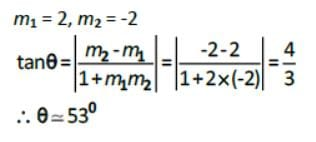Test: Straight Lines- 2 - Question 20

The image of the point (-1 , 2) in the origin is

Test: Straight Lines- 2 - Question 21

The orthogonal projection of the point (2 , - 3) on the line x + y = 0 is

Detailed Solution for Test: Straight Lines- 2 - Question 21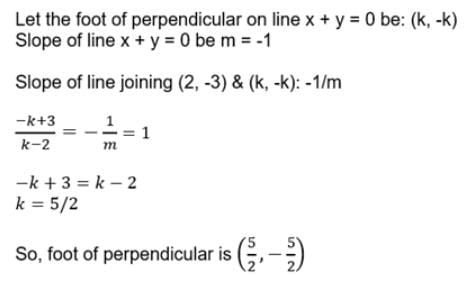Test: Straight Lines- 2 - Question 22

Three points A , B and C are collinear if the area of triangle ABC is

Test: Straight Lines- 2 - Question 23

The lines 8x + 4y = 1, 8x + 4y = 5, 4x + 8y = 3, 4x + 8y = 7 form a

Test: Straight Lines- 2 - Question 24

The number of points on X axis which are at a distance of c units (c < 3) from (2 , 3) is

Test: Straight Lines- 2 - Question 25

If (x , y) are the coordinates of point in the plane , then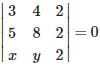represents

## Mathematics (Maths) Class 11

156 videos|176 docs|132 tests
 Use Code STAYHOME200 and get INR 200 additional OFF Use Coupon Code
Information about Test: Straight Lines- 2 Page
In this test you can find the Exam questions for Test: Straight Lines- 2 solved & explained in the simplest way possible. Besides giving Questions and answers for Test: Straight Lines- 2, EduRev gives you an ample number of Online tests for practice

## Mathematics (Maths) Class 11

156 videos|176 docs|132 tests